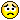0
|
4443 Views
|
4 Replies
|
2 Total Likes
View groups...
Share
GROUPS:

# Problem with integration of gradient function

Posted 10 years ago
 Hi,I want to integrate the sum of the squared gradient of the function   {x, y, z}*Tanh[Norm[{x, y, z}]/2]/Norm[{x, y, z}]so i wroteIntegrate[1/2*(Total[Total[     Evaluate@Grad[        Simplify[          {x, y, z}*Tanh[Norm[{x, y, z}]/2]/Norm[{x, y, z}],         {x, y, z} \[Element] Reals],        {x, y, z}]^2]]), {x, -Infinity, Infinity}, {y, -Infinity, Infinity}, {z, -Infinity, Infinity}]but Integrate will throw warnings at meRefine::cas: Warning: contradictory assumption(s) x\Reals&&y\Reals&&(x!=0||y!=0)&&(Sqrt[-x^2-y^2]!=0||Sqrt[-x^2-y^2]\Reals) encountered. >>Refine::cas: Warning: contradictory assumption(s) x\Reals&&y\Reals&&(x!=0||y!=0)&&(Sqrt[-x^2-y^2]!=0||Sqrt[-x^2-y^2]\Reals) encountered. >>Refine::cas: Warning: contradictory assumption(s) x\Reals&&y\Reals&&(x!=0||y!=0)&&(Sqrt[-x^2-y^2]!=0||Sqrt[-x^2-y^2]\Reals) encountered. >>General::stop: Further output of Refine::cas will be suppressed during this calculation. >>and after literally several HOURS of computing it will return...nothing at all.Can somebody tell me what's going wrong here ?Thanks in advance.
4 Replies
Sort By:
Posted 10 years ago
 Transforming the problem into spherical coordinates would definetly make sense and I'm sure Mathematica has some way of doing the conversion from cartesian to spherical coordinates for me, but I havn't really looked into that, yet.Thank you very much for all the infoPosted 10 years ago
 I am guessing the reason it is so slow is because you are asking it to integrate something relatively complicated and it is just grinding away trying to get there.I may have simplified the integrand somewhat more than you did and that may or may not make it faster.It is possible that the integrand is just complicated enough that it may not be able to figure out that it is infinite, or it may just take longer than you are willing to wait.Looking at the symmetry in my Out, is there any chance that turning this into a problem in spherical coordinates (and figuring out for yourself how to do integration in spherical coordinates) that this problem might be sufficiently simpler that Mathematica could find a solution in a reasonable time?ThisIntegrate[((-1 + r^2 + Cosh[2 r]) Sech[1/2 r]^4)/(8 (r^2)), {r, 0, Infinity}]finishes very quickly, just returning the unchanged integral because it can't find a closed form solution.But I think I need to put a Jacobian in there and it has been long enough that I can't remember exactly how to do that.I could easily be completely confused about this. Make certain that this makes sense before using it.
Posted 10 years ago
 ah nice, thank you very much.yes, i imagined the integral could yield infinite, but wasn't sure. so is this infinity also the reason for the extremely slow behaviour of Integrate call ? Can't it figure out that it is infinite ?
Posted 10 years ago
 If I haven't broken or misunderstood anything then In:= $Assumptions = {x, y, z} \[Element] Reals; f = Simplify[1/2*Total[Total[Grad[Simplify[{x, y, z}*Tanh[Norm[{x, y, z}]/2]/Norm[{x, y, z}]], {x, y, z}]^2]]] Out= ((-1 + x^2+y^2+z^2 + Cosh[2 Sqrt[x^2+y^2+z^2]]) Sech[1/2 Sqrt[x^2+y^2+z^2]]^4)/(8 (x^2+y^2+z^2)) In:= Integrate[f, {x, -Infinity, Infinity}, {y, -Infinity, Infinity}, {z, -Infinity, Infinity}] Out=$Aborted In:= Table[{10^q, NIntegrate[f, {x, -10^q, 10^q}, {y, -10^q, 10^q}, {z, -10^q, 10^q}]}, {q, 1, 4}]Out= {  {10, 131.052},  {100, 1512.39},  {1000, 15325.8},  {10000, 153482.}}then I think your integral might be headed for infinity.I did not wait for hours, but I was unable to reproduce any of your errors.